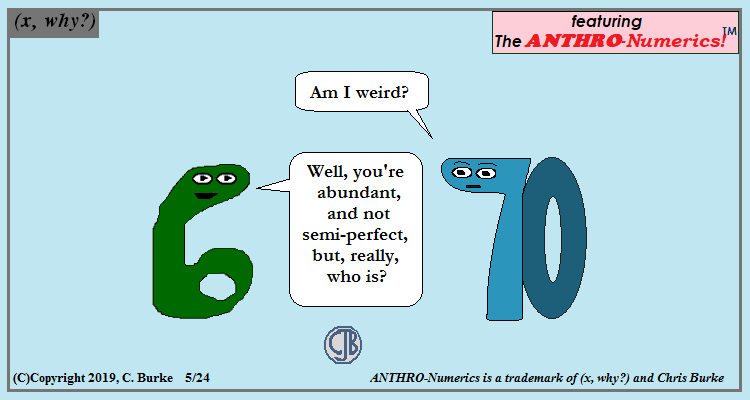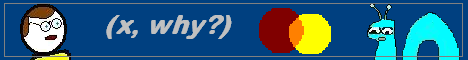## Friday, May 24, 2019

### Weird

(Click on the comic if you can't see the full image.)So, yes, you're weird!

Weird numbers are a subset of Abundant numbers.
In brief:
A perfect number is one where the sum of the number's factors, excluding the number itself, equal the number. Ex: 1+2+3 = 6.
An abundant number is one where the sum of the number's factors, excluding the number itself, is greater than the number. Ex: 1+2+3+4+6=16 > 12.
A semiperfect number is one where a subset of the number's factors have a sum equal to the number. Ex: 1+2+3+6 = 12.
A perfect number is also considered to be semiperfect, unlike my wife who is perfect and I would never consider to be semiperfect.
A weird number is abundant but not semiperfect: there is no subset of factors that add up to the number.
Ex: no combination of 1, 2, 5, 7, 10, 14, and 35 add up to 70, but the sum of the factors is 74.

I was familiar with semiperfect, but not the "weird" term until I was looking up what the prefixes for "abundant" numbers were.

Come back often for more funny math and geeky comics.